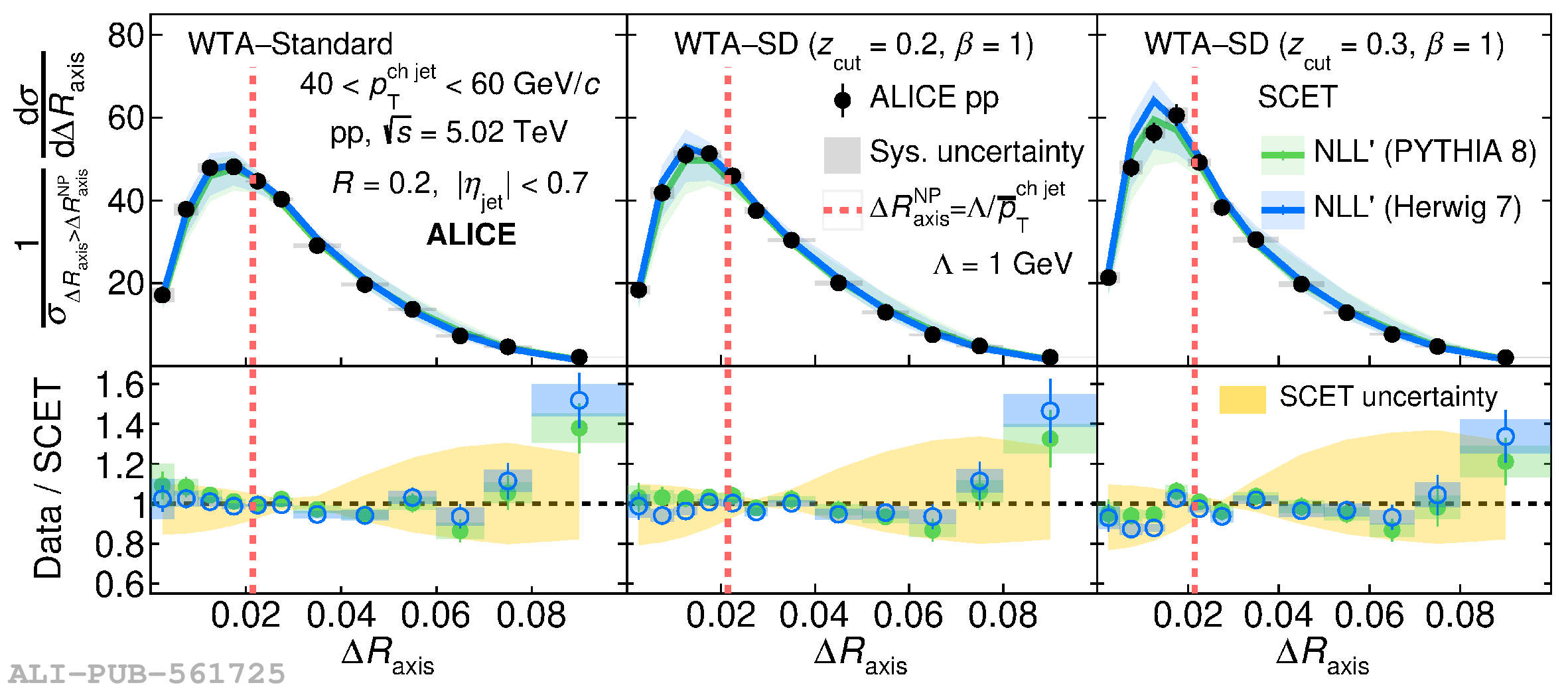# Figure 5

 Comparison between measured distributions and analytic predictions for the difference between the WTA axis and the Standard (left), SD ($\zcut=0.2,\beta=1$) (center), and SD ($\zcut=0.3,\beta=1$) (right) axes for jets of $R=0.4$ (top) and 0.2 (bottom) in the transverse momentum range $40 < \pTchjet < 60$ \GeVc. The black markers correspond to the distributions determined from measured data. The vertical error bars correspond to the statistical uncertainties, and the rectangles correspond to the total systematic uncertainties. The colored curves correspond to the SCET-based analytic predictions corrected for charge and MPI effects using two event generators (PYTHIA 8 and Herwig 7). The vertical dashed line defines the approximate boundary between the non-perturbative and perturbative regions. Both the measured and analytic predictions are normalized so that the integral $\int_{\DeltaR^{\rm NP}}^{R/2}{\rm d}\DeltaR ({\rm d}\sigma/{\rm d}\DeltaR)=1$. The bottom panels show the data/SCET ratios. The colored rectangles correspond to the systematic uncertainty of the measured distribution. The size of the theoretical uncertainty in the analytic predictions is shown as a yellow band.